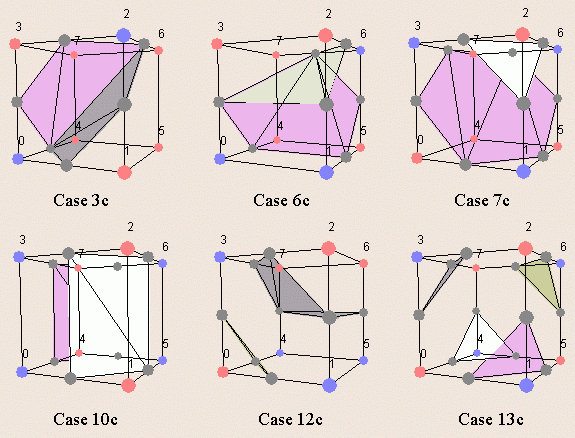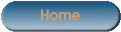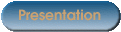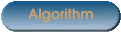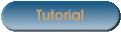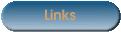As you can see in the previous pages of the site, the marching cubes algorithm is a 3D iso-surface representation technique. In order to explain this technique, we are going to indroduce the marching squares algorithm which uses the same approach in 2D. After having presented some marching squares ambiguous cases we will be able to describe the marching cubes algorithm and an ambiguous case resolution.

# The marching squares algorithm

The marching squares algorithm aims at drawing lines between interpolated values along the edges of a square, considering given weights of the corners and a reference value. Let's consider a 2D grid as shown in the next picture.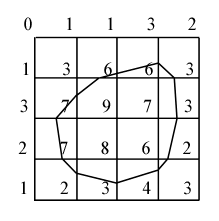Each point of this grid has a weight and here the reference value is known as 5. To draw the curve whose value is constant and equals the reference one, different kinds of interpolation can be used. The most used is the linear interpolation.
In order to display this curve, different methods can be used. One of them consists in considering individually each square of the grid. This is the marching square method.For this method 16 configurations have been enumerated, which allows the representation of all kinds of lines in 2D space.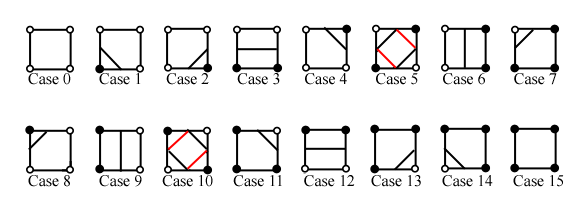# The marching squares ambiguous cases

While trying this algorithm on different configurations we realise that some cases may be ambiguous. That is the situation for the squares 5 and 10.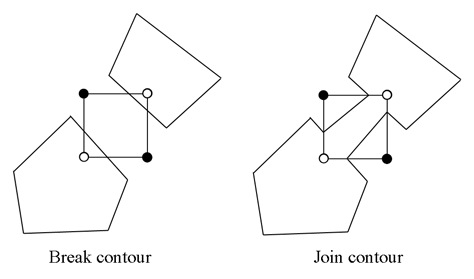As you can see on the previous picture we are not able to take a decision on the interpretation of this kind of situation. However, these exceptions do not imply any real error because the edges keep closed.

# The marching cubes algorithm

Following the marching squares algorithm we can adapt our approach to the 3D case : this is the marching cubes algorithm. In a 3D space we enumerate 256 different situations for the marching cubes representation. All these cases can be generalized in 15 families by rotations and symetries :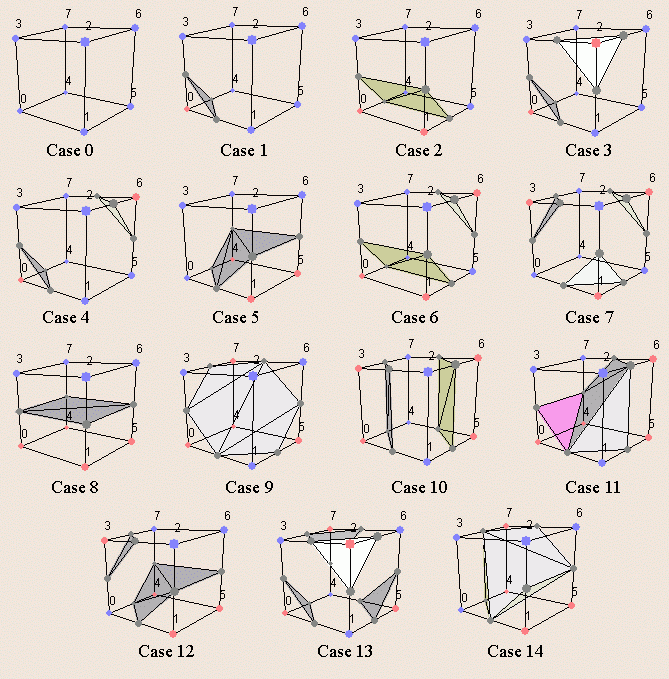In order to be able to determine each real case, a notation has been adopted. It aims at refering each case by an index created from a binary interpretation of the corner weights.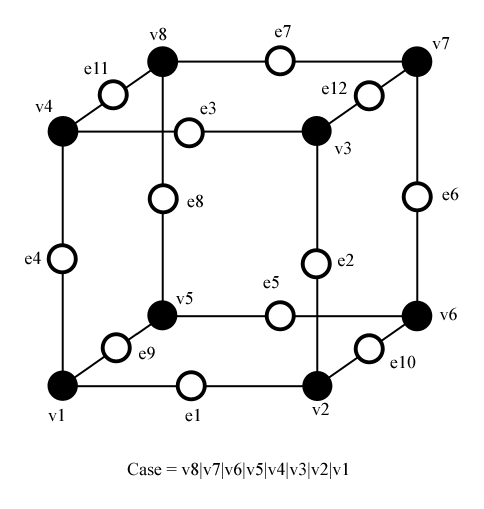In this way, vertexes from 1 to 8 are weighted from 1 to 128 (v1 = 1, v2 = 2, v3 = 4, etc.); for example, the family case 3 example you can see in the picture above, corresponds to the number 5 (v1 and v3 are positive, 1 + 4 = 5).

To all this cubes we can attribute a complementary one. Building a complementary cube consists in reversing normals of the original cube. In the next picture you can see some instances of cubes with their normals.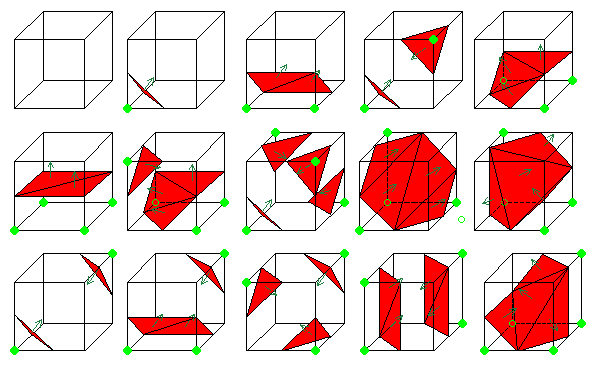The creation of these complementary cubes allows to give an orientation to the surface.

# The marching cubes ambiguous cases

As for the marching squares we meet some ambiguous marching cubes cases : for the family 4 and 10. But in 3D space a solution has to be found because of the topology problems these situations create. However not only real ambiguous cases can create topology disfunctions, some classical families are incompatible. See for instance the next picture.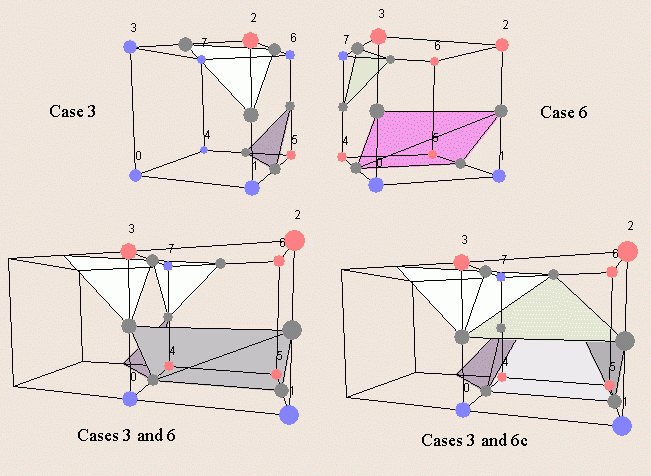To cope with these topology errors (as holes in the 3D model), 6 families have been added to the marching cubes cases. These families have to be used as complementary cases. For instance, in the previous picture, you have to use the case 6c instead of the standard complementary of the case 6.